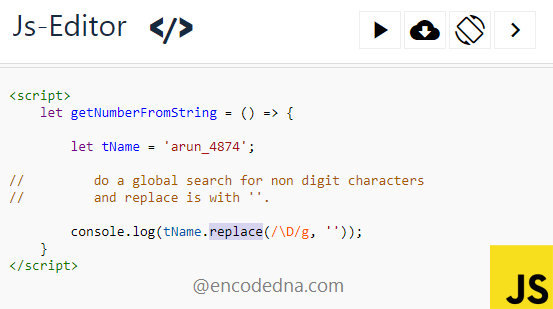﻿ How to Get Numbers from a String in JavaScript

# How to Get Numbers from a String in JavaScript

There are many ways you can extract or get numbers of a string in JavaScript. One of the simplest ways of extracting numbers from a given string in JavaScript is using Regex or Regular Expressions.Let us assume I have a string value, a name, suffixed with some random numbers.

var tName = 'arun_4874';

I want to extract the number 4874 from the above string and there are two simple methods to this.

## 1) Using RegEx \d Metacharacter

```<script>
var tName = 'arun_4874';
</html>```

The metacharacter \d search for digits, which are also numbers. The match() method uses regular expressions to retrieve it results. When used the match() with \d, it returns the number 4874.

However, the above method will return the first occurrence of the numbers. Therefore, if you have a string like arun_4874_541, using the above method, will return only 4874.

To get all the numbers 4874 and 541, you’ll have to use g modifier, which means global (or perform a global search). Here’s how you should do this.

```<script>
var tName = 'arun_4874_541';
</html>```

## 2) Using RegEx \D Metacharacter

Metacharacters are case sensitive and has unique meanings. In the first example above, I have used \d (lower case) and now I’ll use \D upper case.

```<script>
var tName = 'arun_4874';
alert(tName.replace(/\D/g, ''));   // do a global search for non digit characters and replace is with ''.
</html>```

The metacharacter \D (with the g modifier) does a global search for non-digit characters, which returns arun_. And, using the replace() method, I am replacing arun_ with a blank character (''). What remains is the number 4874 and this is our result.

We saw two different metacharacters in action with two different methods, to extract only numbers from a string (any string).

## Extract Numbers from an Element’s id using JavaScript

Here’s another example, where I am extracting numbers from an element’s id and its contents (some text). I am using one of methods that I have described in the above examples.

```<html>
<title>Extract Numbers from String using JavaScript</title>
<body>
<p id='prod21'>
Showing 129 items from our inventory, out of which 6 are sold.
</p>

<input type="button" id="bt1" value="Click it" onclick="getNumberOnly()" />
</body>
<script>
function getNumberOnly() {
var p = document.getElementById('prod21');
var n1 = p.id.match(/\d+/);             // Get numbers from element's id.
var n2 = p.innerHTML.match(/\d+/g);     // Get numbers from element's content.
alert(n1 + ' and ' + n2);
}
</script>
</html>```

Well, that’s it. Thanks for reading.